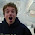## Friday, June 10, 2011

### Looping the Right Way

Many programmers write their loops by starting with a loop over what they have and then producing code to determine what needs to happen for each iteration. However, the result is often code which is hard to read. The underlying problem is that the algorithm they are describing is often naturally implemented by looping over something else. However, the code is awkward as a result of having to adjust for being implemented in a straightforward iteration.

Consider an example of a run length encoder:

`last = Nonecount = 0for letter in input_text:    if letter == last:        count += 1    elif count != 0:        packed = struct.pack(">Bc", count, letter)        output.write(packed)        count = 0    if count == 255:        packed = struct.pack(">Bc", count, letter)        output.write(packed)        count = 0    last = letterif count != 0:    packed = struct.pack(">Bc", count, last)    output.write(packed)`

Following the logic of this code is somewhat difficult. It is also repeats important elements in multiple places. The problem is that the code considers the input letter by letter. However, the run length encoding algorithm really considers the input in blocks of the same character. As a result, the looping logic has become intertwined with the output logic which results in them being harder to read. However, we can easily seperate the two of them.

`blocks = []last = Nonecount = 0for letter in input_text:    if letter == last:        count += 1    elif count != 0:        blocks.append( (letter, count) )        count = 0    if count == 255:        blocks.append( (letter, count) )        count = 0    last = letterif count != 0:    blocks.append( (letter, count) )for letter, count in blocks:    packed = struct.pack(">Bc", count, letter)    output.write(packed)`

Now we've clearly seperated the output and looping logic. The problem with this technique is that it creates a temporary list of blocks. If the data in question is too large this is going to be a problem. However, Python solves this problem with a generator feature. Generator act like lists except that they generate the data as its asked for instead of at the beginning. This also means that you can only iterator once through a generator. Here is an example of the code rewritten to take advantage of that.

`def blocks():    last = None    count = 0    for letter in input_text:        if letter == last:            count += 1        elif count != 0:            yield letter, count            count = 0        if count == 255:            yield letter, count            count = 0        last = letter    if count != 0:        yield letter, countfor letter, count in blocks():    packed = struct.pack(">Bc", count, letter)    output.write(packed)`

However, a further consideration of the blocks function reveals that it does two things. Firstly, it combines repeats of letters. However, it also restricts the size of each block to 255. We can split these into two functions:

`def whole_blocks():    last = None    count = 0    for letter in input_text:        if letter == last:            count += 1        elif count != 0:            yield letter, count            count = 0        last = letter    if count != 0:        yield letter, countdef blocks():    for letter, count in whole_blocks():         while count > 255:              yield letter, 255              count -= 255         yield letter, count`

Now we can actually see the logic more clearly. However, another advantage arises because such small pieces can often be reusable. In fact, whole_blocks can be implemented by using the itertools.groupby method. It divides an iterator up into group of elements which are equal. If we recast the solution in terms of that we get:

`def blocks(count, maxsize = 255):     while count > maxsize:          yield maxsize          count -= maxsize     yield countfor letter, items in itertools.groupby(input_text):    for block_size in blocks(len(list(items))):        packed = struct.pack(">Bc", count, letter)        output.write(packed)`

This code is easier to read and much less error prone then the original code.

The take home lessons:

1. Don't iterate over what you have, decide what you want to iterate over for your algorithm and then figure out how to iterate over that.

2. Divide complex tasks into a number of serials tasks that can be applied to a collection

3. If you find yourself adding flags other variables which change from iteration to iteration, you should look for an alternative

1.2.3.